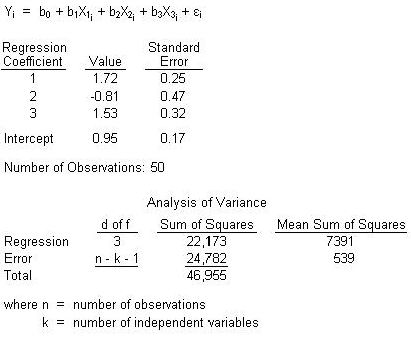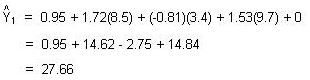### CFA Practice Question

There are 985 practice questions for this topic.

### CFA Practice Question

An analyst is currently reviewing the following regression analysis:If the analyst expects the first, second, and third independent variables to be 8.5, 3.4, and 9.7 respectively, what would be the best estimate of the dependent variable using the regression model?

A. 26.71
B. 27.66
C. 33.16
Explanation:Note, tha hat over the Y implies that this is only an "estimate" of the true value of the dependent variable.